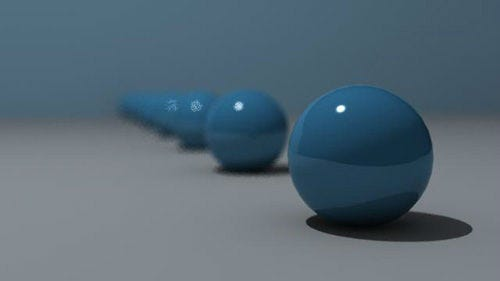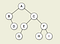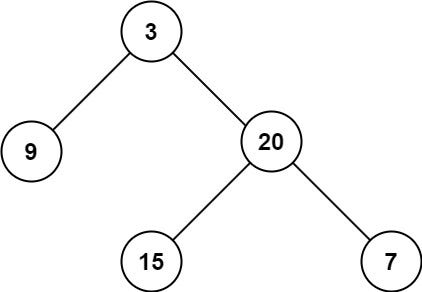# MaxDepth Leetcode Algorithm3DS Max Tutorials — Depth of Field Effect

What does it mean to find the “maximum depth ” of a tree data structure?

Luckily, it is as simple as finding the last remaining french fry at the bottom of your bag! As stated by Leetcode, “A binary tree’s maximum depth is the number of nodes along the longest path from the root node down to the farthest leaf node.tree diagram from StackOverflow

In the diagram above, this tree has a maximum depth of 3!

Where did you get 3 from!?

That’s the question I asked myself when first learning about tree depth. First, you start your count at the root node. Which is A, is our zeroth depth. Moving down the next row of nodes we have B & C, this is where we encounter our first depth. You’ll notice that they have children, so by default, we must keep going down the line to our next depth which is now nodes D, E & F at depth 2. Finally, we come to an end with nodes G, H & I at depth 3. Resulting in this tree’s depth to be a maximum of 3!

Now that we got out of the way, let us solve this leetcode problem with recursion!

Problem: Given the `root` of a binary tree, return its maximum depth.maxDepth — leetcode
`Input: root = [3,9,20,null,null,15,7]Output: 3`

Example 2:

`Input: root = [1,null,2]Output: 2`

Example 3:

`Input: root = []Output: 0`

Example 4:

`Input: root = Output: 1`

Pseudocode:

`function maxDepth(root){(check to see if root is null){return 0 if it does not} otherwise {recursively assign the left nodes to a variablerecursively assign the right nodes to a variableif the left node depth is greater than the right nodes depthreturn either the left otherwise return the right assign the correct node to a childNode variablereturn the childDepth + 1 //this gradually adds to the count of how many depth the tree has}}`

The Solution:

`var maxDepth = function(root) {    if(root == null){        return 0    } else {        let leftDepth = maxDepth(root.left)        let rightDepth = maxDepth(root.right)        var childDepth = leftDepth > rightDepth ? leftDepth : rightDepth;    return 1 + childDepth    }};`

## More from Akiko Green

Software Engineer